# Area of shape + area - math problems

#### Number of problems found: 863

• Rectangular field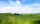What is the area of a rectangular field 100m by 8m?
• Area of a triangle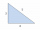Find the area of a triangle with a base of 7 mm and a height of 10 mm?
• 144 stepsTo get around the garden we have to take 144 steps. 8 steps less in width than in length. One step is 5 decimetres long. What are the dimensions of the garden? What is its perimeter and its area?
• The widthThe width of a rectangular garden is 4 m less than the length. If the area of a rectangular garden is 96 square meters, what is the dimension of the garden?
• Circle inscribed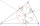There is a triangle ABC and a circle inscribed in this triangle with radius 15. The point T is the point of contact of the inscribed circle with the side BC. What is the area of the triangle ABC if | BT | = 25 a | TC | = 26?
• Semicircle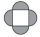The ornament consists of one square and four dark semicircles. The area of the square is 4 cm ^ 2. Find the area of one dark semicircle and round the result to hundreds.
• The isoscelesThe isosceles trapezoid ABCD has bases of 18 cm and 12 cm. The angle at apex A is 60°. What is the circumference and content of the trapezoid?
• Touch circle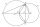Point A has a distance IA, kl = 10 cm from a circle k with radius r = 4 cm and center S. Calculate: a) the distance of point A from the point of contact T if the tangent to the circle is drawn from point A b) the distance of the contact point T from the l
• AlexandraAlexandra made a rectangular quilt the measured 3 1/4. 2 3/4 feet in width. What is the area of the quilt in square feet? Write an equation to solve.
• A rectangle 7A rectangle that has been split into 24 parts, set in 4 rows of 6 parts each. The parts in the top 3 rows have been shaded blue. The parts in the left 5 columns have been shaded purple. The first 5 parts in the top 3 rows are striped to show they are shad
• ParallogramThe Parallelogram base is 24 cm and high 10 cm. How many are such tiles required to cover a floor of area 1080m2?
• A trapezoid 3A trapezoid ABCD has the bases length of a = 120 mm, c = 86 mm and the area A = 2,575 mm2. Find the height of the trapezoid.
• TrapezoidsIn the isosceles trapezoid ABCD we know: AB||CD, |CD| = c = 8 cm, height h = 7 cm, |∠CAB| = 35°. Find the area of the trapezoid.
• A rectangular 4A rectangular garden has a length of 57 m and a width of 42 m. Calculate of how many m2 will decrease the area of a garden, if the ornamental fence with a width of 60 cm will be planted inside its perimeter.
• Perimeter to area 2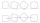Find the area of a square whose perimeter is 100 cm with solve.
• Long and wideWhat is the area of a rectangle that is 1 1/4 meters long and 3/4​ of a meter wide
• A piece 3A piece of rectangle cloth has an area of 2 4/5 meters. Its breadth is 1 1/5 meters what is the length of the cloth?
• Picture 2Picture free is of length 14.2 cm and breadth 10.4 cm. The frame has border of uniforms with 2.6 cm. Find the area of photograph.
• The area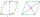The area of a rhombus is 143 m2. If the longer diagonal is 26 m, find the shorter diagonal in inches named d1.
• Cups of paintI have a board that is 2 meters wide and 3 meters tall. If one cup of paint cover 1,000 square centimeters. How many cups of paints does I need?

Do you have an exciting math question or word problem that you can't solve? Ask a question or post a math problem, and we can try to solve it.

We will send a solution to your e-mail address. Solved examples are also published here. Please enter the e-mail correctly and check whether you don't have a full mailbox.

Please do not submit problems from current active competitions such as Mathematical Olympiad, correspondence seminars etc...

Examples of area of plane shapes. Area - math problems.